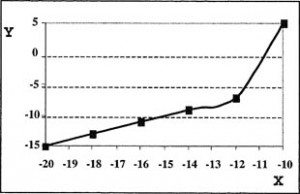## Question 1

Consider the following pattern of numbers:

110, 112, 107, 109, 104
What is the next number in the pattern?

1. 97
2. 99
3. 106
4. 109
3 — 106

## Question 2

Consider the following formula:

A = B +3(4 – C)

If B equals 5 and C equals 2, what is the value of A?

1. 7
2. 11
3. 12
4. 17
2 — 11

## Question 3

Consider the following table:

 X Y 0 -5 1 -4 2 -3 3 -2 4 -1 5 0 6 1

Which of the following choices represents the same relationship as demonstrated in this table?

1.  X Y 10 -40 20 -30 30 -20 40 -10 4 -1 5 0 6 1
2. Y = x/2 – 5
3. Y is equivalent to the difference between the value of X and constant C, where C equals 5.
4.3 — Y is equivalent to the difference between the value of X and constant C, where C equals 5.

## Question Four

Consider the following formula:

a = (1/2)b – 4

Which of the following statements is true for this fomula?

1. When the value of b is less than 8, a is negative.
2. When the value of b is greater than 8, a is negative.
3. When the value of b is less than 8, a is positive.
4. When the value of b is greater than 4, a is positive.
1 — When the value of b is less than 8, a is negative.

## Question Five

Consider the following formula:

y = 3(x + 5)(x – 2)

Which of the following formulas is equivalent to this one?

1. y = 3x squared + 9x – 30
2. y = x squared + 3x – 10
3. y = 3x squared + 3x – 10
4. y = 3x squared + 3x – 30
1 — y = 3×2 + 9x – 30

This section measures your ability to obtain information from written passages. You will be presented with a passage followed by a number of questions about it. A sample passage is shown below, followed by three sample questions. This passage is shorter than those on the actual test.

Passage
The timing of New Year’s Day has changed with customs and calendars. The Mayan civilization, on what is now called the Yucatan peninsula of Mexico, celebrated the New Year on one of the two days when the noonday sun is directly overhead. In the equatorial regions of the earth, between the Tropics of Cancer and Capricorn, the sun is in this position twice a year, once on its passage southward, and once on its passage northward. At the early Mayan city of Izapa in the southern Yucatan, the overhead date for the sun on its southward passage was August 13. The Mayans celebrated this as the date for the beginning of the New Year. Later at the more northerly Mayan site at Edzna, the corresponding overhead date is July 26. Analyses of Mayan pictorial calendars indicate that they celebrated the New Year on August 13 prior to 150 AD, and on July 26 after that year. This change has been explained by archaeological dating showing that 150 AD was the time that the Mayans moved the hub of their civilization from the southern to the northern site.

## Question 6

According to the passage, the sun at Edzna was directly overhead at noon on:

1. July 26 only
2. August 13 only
3. July 26 and one other date
4. August 13 and one other date
3 — July 26 and one other date

## Question 7

If the Mayans had moved their civilization’s center south of Izapa, their new date for celebration of the New Year would probably have been closest to which of the following dates?

1. January 1
2. February 20
3. March 25
4. September 15